# 3.2. EM1DFM Supporting Files¶

## 3.2.1. Observation File¶

This file contains the observations and all the survey parameters, with five lines including parameters as follows:

1. the number of soundings

2. the x- and y-coordinates of each sounding, and the number of frequencies per sounding

3. each frequency, and the number of transmitters for each frequency

4. the dipole moment of each transmitter, their z-coordinates and orientations, and the number of receivers for each

5. the last line contains all of:

• the dipole moment of each receiver,
• the transmitter-receiver separation in the x- and y-directions,
• the z-coordinate and orientation of each receiver,
• what type of normalization has been applied to the observations,
• whether in-phase and/or quadrature data are present,
• the actual observed field values for this receiver, and
• the type of uncertainties and their values.

The structure of the observations file is as follows. Click on the letter labels for variable names, and for parameter details:

soundx_a $$\;\;$$ soundy_a $$\;\;$$ nfreqs_a
freq_a $$\;\;$$ nt_a
momt_a $$\;\;$$ zt_a $$\;\;$$ ot_a $$\;\;$$ nr_a
momr_a $$\;\;$$ trx_a $$\;\;$$ try_a $$\;\;$$ zr_a $$\;\;$$ or_a $$\;\;$$ ontype_a $$\;\;$$ octype_a $$\;\;$$ obs_a $$\;\;$$ utype_a $$\;\;$$ uncert_a

• $$\mathbf{nsounds}$$ is the number of soundings. Each sounding refers to an independent horizontal location where the Earth may be considered as a layered model. Thus, the EM1DFM code inverts for an independent layered Earth model for each sounding. This is used to infer horizontal variability in the Earth’s conductivity and/or susceptibility structure.
• $$\mathbf{soundx\_ a(i_s)}$$ is the x-coordinate of the $$i_s^{th}$$ sounding
• $$\mathbf{soundy\_ a(i_s)}$$ is the y-coordinate of the $$i_s^{th}$$ sounding
• $$\mathbf{nfreqs \_ a(i_s)}$$ is the number of frequencies for the $$i_s^{th}$$ sounding
• $$\mathbf{freq\_ a(i_f, i_s)}$$ is the frequency (Hz) of the $$i_f^{th}$$ frequency for the $$i_s^{th}$$ sounding
• $$\mathbf{nt\_ a(i_f,i_s)}$$ is the number of transmitters for the $$i_f^{th}$$ frequency for the $$i_s^{th}$$ sounding
• $$\mathbf{momt\_ a(i_t,i_f,i_s)}$$ is the dipole moment (Am $$\! ^2$$) of the $$i_t^{th}$$ transmitter for the $$i_f^{th}$$ for the $$i_s^{th}$$ sounding
• This number is used as a simple scaling within the program. If $$momt_a$$ = 2, then the forward-modelled observations are twice what they would be if $$momt_a$$ = 1.
• $$\mathbf{zt\_ a (i_t,i_f,i_s)}$$ is the z-coordinate (metres, negative upwards from zero on the Earth’s surface) of the $$i_t^{th}$$ transmitter for the $$i_f^{th}$$ frequency for the $$i_s^{th}$$ sounding
• $$\mathbf{ot\_a(i_t,i_f,i_s)}$$ is the orientation of the $$i_t^{th}$$ transmitter for the $$i_f^{th}$$ frequency for the $$i_s^{th}$$ sounding (“x” for an x-directed dipole, “y” for a y-directed dipole, and “z” for a vertical (downward-directed) dipole)
• $$\mathbf{nr\_ a(i_t,i_f,i_s)}$$ is the number of receivers for the $$i_t^{th}$$ transmitter for the $$i_f^{th}$$ frequency for the $$i_s^{th}$$ sounding
• $$\mathbf{momr\_ a(i_t,i_f,i_s)}$$ is a scale factor for the $$i_r^{th}$$ receiver for the $$i_t^{th}$$ transmitter for the $$i_f^{th}$$ frequency for the $$i_s^{th}$$ sounding which allows the incorporation of any necessary parameters of the receiver that might mean the observations are not simply point measurements of the H-field.

• An example of necessary parameters could be coil area and/or number of turns and/or orientation (e.g., $$momr_a$$ = −1 for an upward-pointing z-directed receiver dipole).
• This number simply appears as a scale factor within the code (i.e. if $$momr_a$$ = 2, then the forward-modelled observations are twice what they would be if $$momr_a$$ = 1)
• NOTE: Some common data formats (such as DIGEM coaxial data - not coplanar data) require $$momr_a$$ = -1 to make these data compatible with the normalization convention used by EM1DFM. The first example requires this type of normalization
• $$\mathbf{trx\_ a(i_r,i_t,i_f,i_s)}$$ is the transmitter-receiver separation (m) in the x-direction between the $$i_r^{th}$$ receiver and the $$i_t^{th}$$ transmitter for the $$i_f^{th}$$ frequency for the $$i_s^{th}$$ sounding
• $$\mathbf{try\_ a(i_r,i_t,i_f,i_s)}$$ is the transmitter-receiver separation (m) in the y-direction between the $$i_r^{th}$$ receiver and the $$i_t^{th}$$ transmitter for the $$i_f^{th}$$ frequency for the $$i_s^{th}$$ sounding
• $$\mathbf{zr\_ a(i_r,i_t,i_f,i_s)}$$ is the z-component (metres, negative upwards from zero on the Earth’s surface) of the $$i_r^{th}$$ receiver for the $$i_t^{th}$$ transmitter for the $$i_f^{th}$$ frequency for the $$i_s^{th}$$ sounding
• $$\mathbf{or\_ a(i_r,i_t,i_f,i_s)}$$ is the orientation of the $$i_r^{th}$$ receiver for the $$i_t^{th}$$ transmitter for the $$i_f^{th}$$ frequency for the $$i_s^{th}$$ sounding
• “x” for an x-directed dipole,
• “y” for a y-directed dipole, and
• “z” for a vertical (downward-directed) dipole
• $$\mathbf{ontype\_ a(i_r,i_t,i_f,i_s)}$$ is the type of normalization of the data/datum for the $$i_r^{th}$$ receiver for the $$i_t^{th}$$ transmitter for the $$i_f^{th}$$ frequency for the $$i_s^{th}$$ sounding

• $$ontype\_ a$$ = 1 indicates the data are values in ppm of the secondary magnetic field normalized by the free-space magnetic field
• $$ontype\_ a$$ = 2 indicates the data are values in % of the secondary magnetic field normalized by the free-space magnetic field
• $$ontype\_ a$$ = 3 indicates the data are values of the secondary H-field in A/m, and
• $$ontype\_ a$$ = 4 indicates the data are values of the total H-field in A/m
• $$\mathbf{octype\_ a(i_r,i_t,i_f,i_s)}$$ is the observation type for the $$i_r^{th}$$ receiver for the $$i_t^{th}$$ transmitter for the $$i_f^{th}$$ frequency for the $$i_s^{th}$$ sounding

• $$octype\_ a$$ = “b” indicates both inphase and quadrature observations are present
• $$octype\_ a$$ = “i” just the inphase observation is present
• $$octype\_ a$$ = “q” just the quadrature datum
• $$\mathbf{obs\_ a(i_r,i_t,i_f,i_s)}$$ is the pair of inphase and quadrature observations, or just the in-phase observation, or just the quadrature observation, for the $$i_r^{th}$$ receiver for the $$i_t^{th}$$ transmitter for the $$i_f^{th}$$ frequency for the $$i_s^{th}$$ sounding
• $$\mathbf{utype\_ a(i_r,i_t,i_f,i_s)}$$ indicates the form in which the uncertainties are provided for the $$i_r^{th}$$ receiver for the $$i_t^{th}$$ transmitter for the $$i_f^{th}$$ frequency for the $$i_s^{th}$$ sounding

• $$utype\_ a$$ = “v” for absolute uncertainties in the same units as the observations, and
• $$utype\_ a$$ = “p” percentage uncertainties
• $$\mathbf{uncert\_ a(i_r,i_t,i_f,i_s)}$$ is the pair of uncertainties for the inphase and quadrature observations, or the uncertainty in just the inphase observation, or the uncertainty in just the quadrature observation, for the $$i_r^{th}$$ receiver for the $$i_t^{th}$$ transmitter for the $$i_f^{th}$$ frequency for the $$i_s^{th}$$ sounding

Example for a single sounding

The observations file for a single sounding (that is, a single one-dimensional model) at x = 0 m, y = 0 m for an airborne-type configuration is shown below.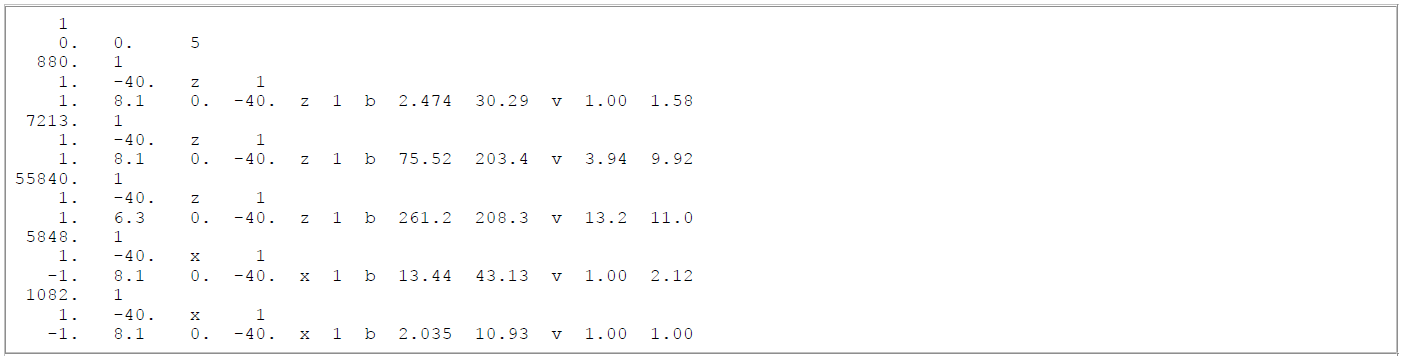• There are three frequencies for the horizontal coplanar loop configuration (880, 7213, 55840 Hz)

• a z-directed magnetic dipole transmitter (dipole moment = 1 Am $$\! ^2$$) and receiver (dipole moment = 1 Am $$\! ^2$$) for each frequency separated by 8.1 m, 8.1 m and 6.3 m respectively in the x-direction, 0 m in the y-direction
• both transmitter and receiver for each frequency are at a height of 40 m above the Earth’s surface
• There are two frequencies for the coaxial loop configuration (5848 and 1082 Hz)

• an x-directed magnetic dipole transmitter (dipole moment = 1 A m2) and receiver (dipole moment = −1 A m2) for both frequencies separated by 8.1 m in the x-direction and 0 m in the y-direction;
• both transmitter and receiver for each frequency are at a height of 40 m above the Earth’s surface.
• The observations are values of the secondary magnetic field normalized by the free-space field and expressed in terms of parts-per-million (ppm).

• Both inphase and quadrature components of the field are supplied.

• The uncertainties are expressed in absolute terms in the same units as the observations (i.e., ppm).

Example for EM-31 data for two soundings

The observations file for EM31-type data for two soundings is shown below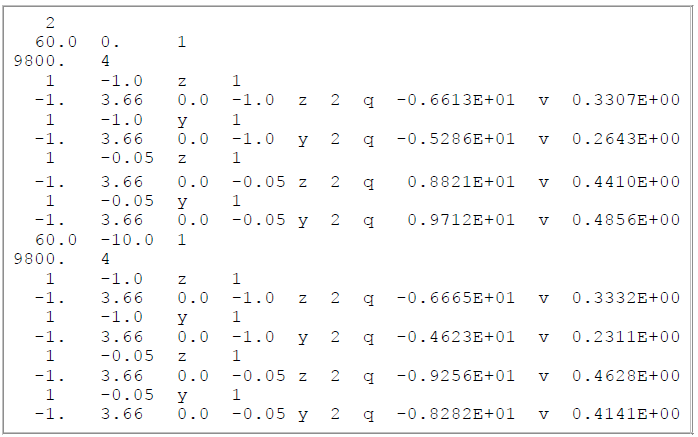• Sounding locations are at x = 60 m & y = 0 m and x = 60 m & y = −10 m.

• There is one frequency (9.6 kHz),

• There are four instrument positions:

• at waist height (1 m) and on the ground (0.05 m),
• held both normally (vertical transmitter and receiver coil axes) and on its side (horizontal coil axes).
• The transmitter and receiver coils are separated by 3.66 m in the x-direction. Only the quadrature part of the normalized secondary H-field (in %) is provided as data,

• The uncertainties are absolute in %.

## 3.2.2. Files for reference and starting models¶

### 3.2.2.1. Conductivity files¶

Required if mtype = 1, 3 or 4 in the input file

This is the file containing the starting conductivity model for all soundings if conductivity is active in the inversion. The relevant quantities are the number of layers, and the thickness (m) and conductivity (S/m) of each layer. A dummy value for the thickness of the basement halfspace is required in this file, but nothing is ever done with it after it is read in. If conductivity is active in the inversion, it is from this file that the program gets the number of layers and their thicknesses, which then must be the same for all other models read in by the program. This file is therefore required for mode mtype = 1, 3 or 4 (i.e. conductivity is active in the inversion). This file can also be a layers-only file to indicate that the best-fitting halfspace is to be used as the starting model.

The structure of this file is as follows (just as for all 1D model files). For a layers-only file the conductivity column is left blank: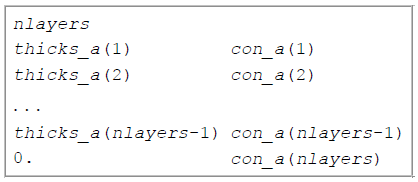Fig. 3.1 File structure for conductivity models

• nlayers is the number of layers in the model,
• thicks_a(j) is the thickness in metres of the jth layer and
• con_a(j) is the conductivity in S/m of the jth layer.

An example file for a conductivity model made up of 12 layers (including the basement halfspace) is shown below. The thicknesses of the first eleven layers increase from 4.7987 m to 135.76 m. This model is a homogeneous halfspace of 0.3 milliSeimens per m.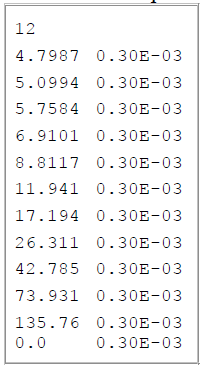Fig. 3.2 Conductivity file example

### 3.2.2.2. Susceptibility files¶

Required if mtype = 2 in the input file

This file contains the starting susceptibility model for all soundings if susceptibility is active in the inversion. The file contains the number of layers, and the thickness (m) and susceptibility (SI units) of each layer. A dummy value for the thickness of the basement halfspace is required, but nothing is done with it after it is read in.

If only susceptibility is active (i.e., mtype = 2), the inversion program gets it’s information about the number of layers in the model and their thicknesses from this file. If both conductivity and susceptibility are active (i.e., mtype = 3 or 4), the program gets the number of layers and their thicknesses from the starting conductivity model - see above. All other models (e.g., reference models) read in must then have the same number of layers with exactly the same thicknesses.

The structure of this file is as follows (just as for all 1D model files). For a layers-only file the susceptibility column is left blank: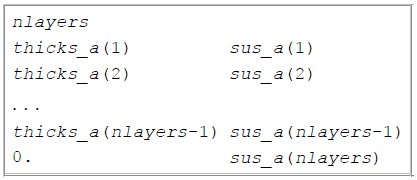Fig. 3.3 File structure for susceptibility models

• nlayers is the number of layers in the model,
• thicks_a(j) is the thickness in metres of the jth layer and
• sus_a(j) is the susceptibility in SI units of the jth layer.

### 3.2.2.3. Other inputs for starting and reference models¶

File for reference conductivity model (for smallest model component) (Optional)

The parameter describing the reference conductivity model for the smallest component of the model norm if one is required for the inversion, or describing the reference conductivity model if only susceptibility is active in the inversion. The parameter is either “NONE”, OR a file OR a value for a halfspace OR “default” if the best-fitting halfspace is to be used. It is required if mtype = 1, 3 or 4 and acs > 0, or if mtype = 2. If a file is used, it must have the same format as the starting conductivity model file (see above), and it must have the same number of layers with exactly the same thicknesses as the starting conductivity and/or susceptibility model.

File for reference susceptibility model (for smallest model component) (Optional)

The parameter describing the reference susceptibility model for the smallest component of the model norm if one is required for the inversion, or describing the reference susceptibility model if only conductivity is active in the inversion. That is, this model is required if mtype = 2, 3 or 4 and ass > 0, or if mtype = 1. The parameter is either “NONE”, OR a file OR a value for a halfspace OR “default” if the best-fitting halfspace is to be used. If a file is used, it must be in the same format as all model files (see above), and must have the same number of layers with exactly the same thicknesses as the starting conductivity and/or susceptibility model.

File for reference conductivity model (for flattest model component) (Optional - not available in the GUI)

The parameter describing the reference conductivity model for the flattest component of the model norm if one is required for the inversion. Whether or not this parameter is specified in “em1dfm.in” determines whether or not such a reference model plays a part in the inversion. The parameter is either “NONE”, OR a file OR a value for a halfspace OR “default” if the best-fitting halfspace is to be used. If a file is used, it must be in the same format as the starting conductivity model file (see section above), and must have the same number of layers with exactly the same thicknesses as the starting conductivity and/or susceptibility model.

File for reference susceptibility model (for flattest model component) (Optional - not available in the GUI)

The parameter describing the reference susceptibility model for the flattest component of the model norm if one is required for the inversion. Whether or not this parameter is specified in “em1dfm.in” determines whether or not such a reference model plays a part in the inversion. The parameter is either “NONE”, OR a file OR a value for a halfspace OR “default” if the best-fitting halfspace is to be used. If a file is used, it must be in the same format as the starting susceptibility model file (see section above), and must have the same number of layers with exactly the same thicknesses as the starting conductivity and/or susceptibility model.

## 3.2.3. File for additional model-norm weights¶

The file containing the information about the additional weighting of the layers for some or all of the four possible components of the model norm: smallest and flattest components for conductivity and susceptibility.

• The first line of this file must contain two (if mtype = 1 or 2) or four (if mtype = 3 or 4) integers (which can either have the value 0 or 1) to indicate that weights are being supplied for use in the two or four components of the model norm
• e.g., “1 0” for mtype = 1 implies that additional weights are supplied for use in the smallest component of the model norm but not the flattest component for only conductivity active in the inversion;
• “1 0” for mtype = 2 implies that additional weights are supplied for use in the smallest component of the model norm but not the flattest component for only susceptibility active in the inversion;
• “1 0 1 0” for mtype = 3 or 4 implies that additional weights are supplied for both the smallest component of the conductivity portion of the model norm and the smallest component of the susceptibility portion of the model norm, but not for the flattest components, when both conductivity and susceptibility are active in the inversion.
• The second line of this file must contain the number of layers in the model. The order of the four possibilities must be the same as shown below, with any set of weights that is not needed by the program simply omitted.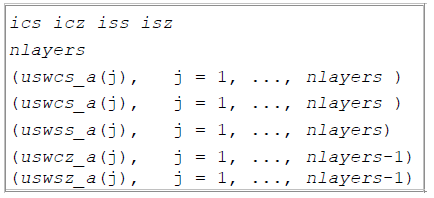The parameters within the this file are described as follows:

• ics, icz, iss & isz are the four integers that indicate the presence of weights for the smallest and flattest components of the model norm for conductivity and the smallest and flattest components of the model norm for susceptibility (if mtype = 2,ics & icz are omitted),
• nlayers is the number of layers in the model;
• uswcs_a(j) is the weight for the jth layer in the smallest component of the conductivity portion of the model norm;
• uswss_a(j) is the weight for the jth layer in the smallest component of the susceptibility portion of the model norm;
• uswcz_a(j) is the weight for the difference between the jth and (j+1)th layers in the flattest component of the conductivity component of the model norm; and
• uswsz_a(j) is the weight for the difference between the jth and (j+1)th layers in the flattest component of the susceptibility component of the model norm.

The supplied weights must be greater than zero. A weight greater than one increases the weight relative to the default setting, and a weight less than one decreases the weight relative to the default setting